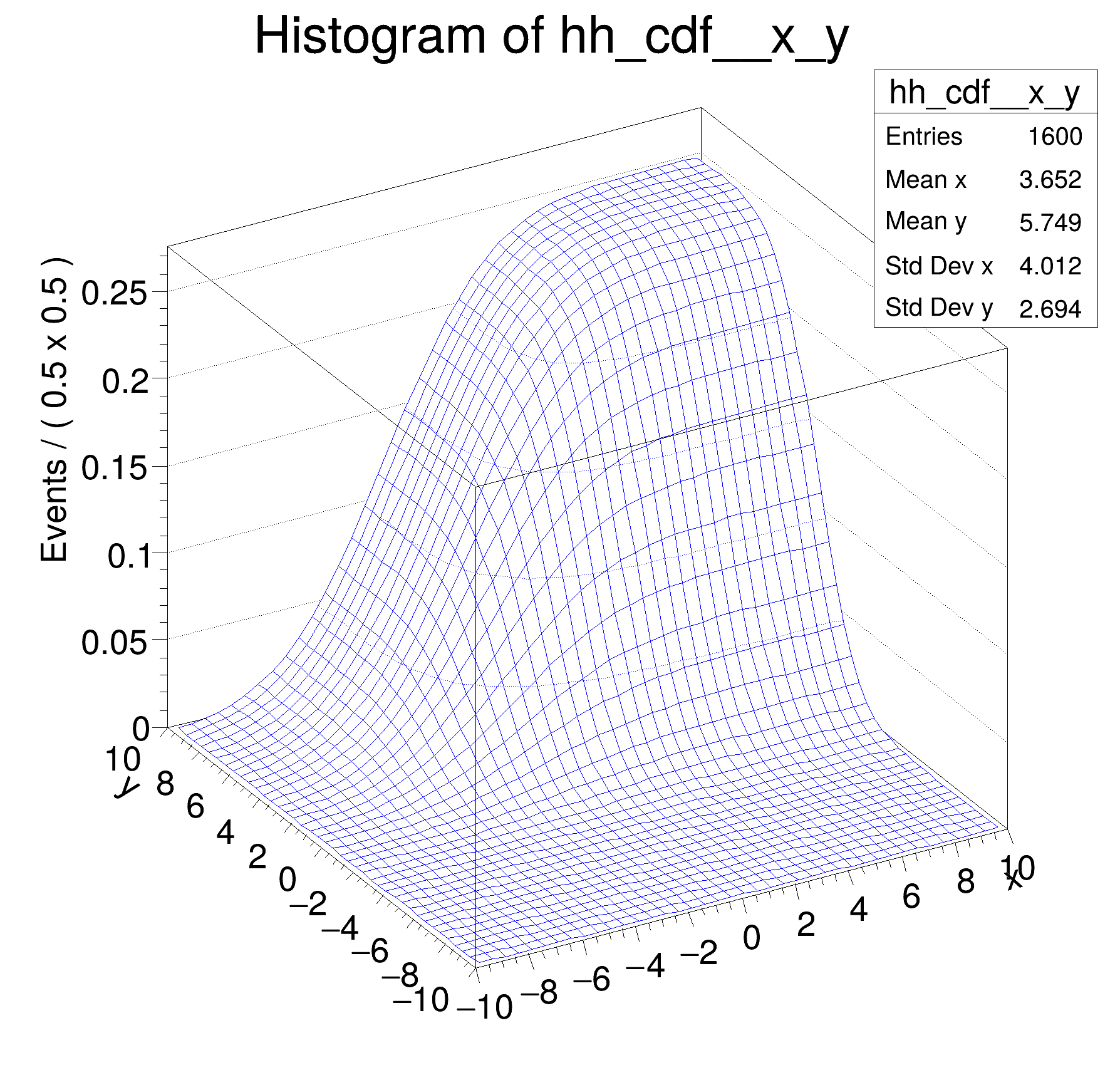ROOT   Reference Guide
Searching...
No Matches
rf308_normintegration2d.C File Reference

## Detailed DescriptionMultidimensional models: normalization and integration of pdfs, construction of cumulative distribution functions from pdfs in two dimensions#include "RooRealVar.h"
#include "RooGaussian.h"
#include "RooProdPdf.h"
#include "RooAbsReal.h"
#include "RooPlot.h"
#include "TCanvas.h"
#include "TAxis.h"
#include "TH1.h"
using namespace RooFit;
{
// S e t u p m o d e l
// ---------------------
// Create observables x,y
RooRealVar x("x", "x", -10, 10);
RooRealVar y("y", "y", -10, 10);
// Create pdf gaussx(x,-2,3), gaussy(y,2,2)
RooGaussian gx("gx", "gx", x, -2.0, 3.0);
RooGaussian gy("gy", "gy", y, +2.0, 2.0);
// Create gxy = gx(x)*gy(y)
RooProdPdf gxy("gxy", "gxy", RooArgSet(gx, gy));
// R e t r i e v e r a w & n o r m a l i z e d v a l u e s o f R o o F i t p . d . f . s
// --------------------------------------------------------------------------------------------------
// Return 'raw' unnormalized value of gx
cout << "gxy = " << gxy.getVal() << endl;
// Return value of gxy normalized over x _and_ y in range [-10,10]
RooArgSet nset_xy(x, y);
cout << "gx_Norm[x,y] = " << gxy.getVal(&nset_xy) << endl;
// Create object representing integral over gx
// which is used to calculate gx_Norm[x,y] == gx / gx_Int[x,y]
std::unique_ptr<RooAbsReal> igxy{gxy.createIntegral(RooArgSet(x, y))};
cout << "gx_Int[x,y] = " << igxy->getVal() << endl;
// NB: it is also possible to do the following
// Return value of gxy normalized over x in range [-10,10] (i.e. treating y as parameter)
RooArgSet nset_x(x);
cout << "gx_Norm[x] = " << gxy.getVal(&nset_x) << endl;
// Return value of gxy normalized over y in range [-10,10] (i.e. treating x as parameter)
RooArgSet nset_y(y);
cout << "gx_Norm[y] = " << gxy.getVal(&nset_y) << endl;
// I n t e g r a t e n o r m a l i z e d p d f o v e r s u b r a n g e
// ----------------------------------------------------------------------------
// Define a range named "signal" in x from -5,5
x.setRange("signal", -5, 5);
y.setRange("signal", -3, 3);
// Create an integral of gxy_Norm[x,y] over x and y in range "signal"
// This is the fraction of of pdf gxy_Norm[x,y] which is in the
// range named "signal"
std::unique_ptr<RooAbsReal> igxy_sig{gxy.createIntegral({x, y}, NormSet(RooArgSet(x, y)), Range("signal"))};
cout << "gx_Int[x,y|signal]_Norm[x,y] = " << igxy_sig->getVal() << endl;
// C o n s t r u c t c u m u l a t i v e d i s t r i b u t i o n f u n c t i o n f r o m p d f
// -----------------------------------------------------------------------------------------------------
// Create the cumulative distribution function of gx
// i.e. calculate Int[-10,x] gx(x') dx'
std::unique_ptr<RooAbsReal> gxy_cdf{gxy.createCdf(RooArgSet(x, y))};
// Plot cdf of gx versus x
TH1 *hh_cdf = gxy_cdf->createHistogram("hh_cdf", x, Binning(40), YVar(y, Binning(40)));
hh_cdf->SetLineColor(kBlue);
new TCanvas("rf308_normintegration2d", "rf308_normintegration2d", 600, 600);
hh_cdf->GetZaxis()->SetTitleOffset(1.8);
hh_cdf->Draw("surf");
}
@ kBlue
Definition Rtypes.h:66
RooArgSet is a container object that can hold multiple RooAbsArg objects.
Definition RooArgSet.h:55
Plain Gaussian p.d.f.
Definition RooGaussian.h:24
RooProdPdf is an efficient implementation of a product of PDFs of the form.
Definition RooProdPdf.h:33
RooRealVar represents a variable that can be changed from the outside.
Definition RooRealVar.h:37
virtual void SetTitleOffset(Float_t offset=1)
Set distance between the axis and the axis title.
Definition TAttAxis.cxx:298
virtual void SetLineColor(Color_t lcolor)
Set the line color.
Definition TAttLine.h:40
The Canvas class.
Definition TCanvas.h:23
TH1 is the base class of all histogram classes in ROOT.
Definition TH1.h:58
TAxis * GetZaxis()
Definition TH1.h:324
void Draw(Option_t *option="") override
Draw this histogram with options.
Definition TH1.cxx:3063
RooCmdArg YVar(const RooAbsRealLValue &var, const RooCmdArg &arg={})
RooCmdArg NormSet(Args_t &&... argsOrArgSet)
RooCmdArg Binning(const RooAbsBinning &binning)
Double_t y[n]
Definition legend1.C:17
Double_t x[n]
Definition legend1.C:17
The namespace RooFit contains mostly switches that change the behaviour of functions of PDFs (or othe...
Ta Range(0, 0, 1, 1)
gxy = 0.485672
gx_Norm[x,y] = 0.0129332
gx_Int[x,y] = 37.5523
gx_Norm[x] = 0.106896
gx_Norm[y] = 0.120989
[#1] INFO:Eval -- RooRealVar::setRange(x) new range named 'signal' created with bounds [-5,5]
[#1] INFO:Eval -- RooRealVar::setRange(y) new range named 'signal' created with bounds [-3,3]
gx_Int[x,y|signal]_Norm[x,y] = 0.572035
Date
July 2008

Definition in file rf308_normintegration2d.C.